### Home > AC > Chapter 14 > Lesson 14.3.1.3 > Problem3-46

3-46.
1. Examine each sequence below. State whether it is arithmetic, geometric, or neither. For the sequences that are arithmetic, find the formula for t(n). For the sequences that are geometric, find the sequence generator for t(n). Homework Help ✎

1. 1, 4, 7, 10, 13, …

2. 0, 5, 12, 21, 32, …

3. 2, 4, 8, 16, 32, …

4. 5, 12, 19, 26, …

5. x, x + 1, x + 2, x + 3, …

6. 3, 12, 48, 192, …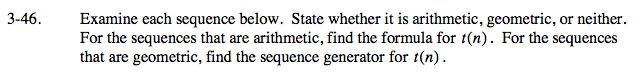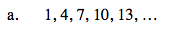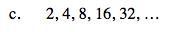The sequence is geometric.
r = 2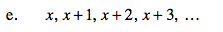See part (d).

The sequence is arithmetic.
d = 1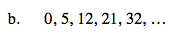The sequence is neither arithmetic nor geometric.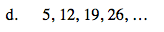Do the terms in the sequence increase by adding a constant amount or multiplying by a constant?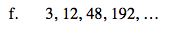See part (d).